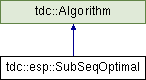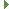tudocomp – The TU Dortmund Compression Framework
tdc::esp::SubSeqOptimal Class Reference

#include <SubseqStrategy.hpp>

Inheritance diagram for tdc::esp::SubSeqOptimal:Public Member Functions

template<typename SortedIndices >
Dpi_and_b create_dpi_and_b_from_sorted_indices (const SortedIndices &sorted_indices) constPublic Member Functions inherited from tdc::Algorithm
virtual ~Algorithm ()=default

Algorithm (Algorithm const &)=default

Algorithm (Algorithm &&)=default

Algorithmoperator= (Algorithm const &)=default

Algorithmoperator= (Algorithm &&)=default

Algorithm (Env &&env)
Instantiates an algorithm in the specified environment. More...

Envenv ()

const Envenv () const

Static Public Member Functions

static Meta meta ()

Detailed Description

Definition at line 8 of file SubseqStrategy.hpp.

◆ create_dpi_and_b_from_sorted_indices()

template<typename SortedIndices >
 Dpi_and_b tdc::esp::SubSeqOptimal::create_dpi_and_b_from_sorted_indices ( const SortedIndices & sorted_indices ) const
inline

Definition at line 18 of file SubseqStrategy.hpp.

◆ meta()

 static Meta tdc::esp::SubSeqOptimal::meta ( )
inlinestatic

Definition at line 10 of file SubseqStrategy.hpp.

The documentation for this class was generated from the following file: